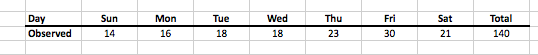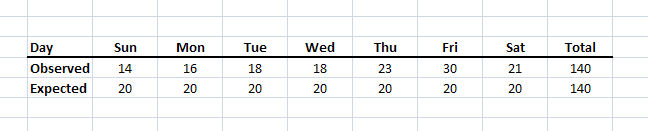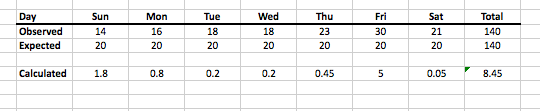### Testing the Significance of Day-of-Week Analysis with the Chi-Square Statistical Test

A common weapon in the crime analyst arsenal is the day-of-week analysis. One problem with the technique is that many people accept the results uncritically and assume that if, for example, there have been several more incidents on Friday than any other day then Friday is the best day for a focused action. The problem is that day-of-week analysis is seldom accompanied by a confidence interval or evaluated to find out if the results are statistically significant. Without performing the statistical follow-up it’s impossible to know if the results actually represent anything other than randomness.

In order to evaluate statistical significance for something like a day-of-week analysis we need to use the Chi-Square Statistical Test. The chi-square test is what’s known as a non-parametric test which is a fancy way to say that it will work with nominal data (that is categories) and that the hypothesis being measured does not require normal distribution or any variance assumptions (a bonus for testing things like a day-of-week breakdown). The test works by performing a goodness-of-fit analysis between the observed data and an expected distribution. The point is to determine if the differences between the observed and expected are caused by something other than chance.

To make things more concrete let me introduce the actual data I will be evaluating. The following image lists some (fictional) motor vehicle collision (MVC) counts over the course of several weeks. Looking at the data with an uncritical eye might lead some to assume that Fridays are the worst day for collisions and that we should do a traffic blitz on that day. The purpose of the exercise is to see if that’s a valid assumption.So this is our “observed data” but what about the “expected distribution”? In order to answer we need to identify another property of the statistical significance test: the null hypothesis. In any statistical test we need two hypotheses: the research hypothesis and the null hypothesis. The research hypothesis is what we’re hoping to show, in this case, that the day of week means something for MVCs. The null hypothesis is the opposite and in our example it means that the day of the week doesn’t factor into when collisions take place. Since the null hypothesis states that the day doesn’t matter then we should expect the same number of collisions each day. If the total number of collisions is 140 then the expected value for each day is 140/7 = 20. With this new info have a look at the following image that lists both the observed and theoretical distributions.The chi-square calculation is pretty straight forward: subtract each observed value from its expected value, square it, and then divide by the expected value. So for Sunday the value would be (14-20)^2/20 = 1.8., for Monday it’s: (16-20)^2/20 = 0.8 and so on for each of the 7 days. Then you sum up each of the calculated values to arrive at a total score (8.45 in this case), as in the image below.The next step is to check the score against what’s known as a chi-square critical value table, which is pre-computed tables of critical values for different confidence intervals and degrees of freedom. The tables are all over the Internet but I used this one for this example. I wrote above about confidence intervals, and I’m going to pick the 95% interval just because, which means that my alpha value (look at the table) is 0.05 (i.e. 1 – 0.95). The second value is degrees of freedom which is a measure of how many elements are being tested, minus one. For this example, 7 days in the week, minus one, means we have 6 degrees of freedom (df). Knowing these two pieces of information we can look up the chi-square critical value. Go down to the row for df=6 and across to the column for alpha=0.05 and the number is 12.592.

12.592 is greater than 8.45. That means we cannot reject the null hypothesis. That means we can’t say that the observed MVCs across the days of the week is due to anything other than chance. It means that we can’t say that any particular day is better than another to do a traffic blitz because, statistically, the observed data could have happened by chance. This is a troubling truth if we’ve been telling officers to focus on Fridays.

So this post is kind of a bummer if you’re used to directing officers based on day-of-week analysis. A lot of officers don’t want to hear about statistical significance (well, actually, none, I don’t know any who would want to hear about it) but the hard truth is that math is telling us that what we think is a pattern (e.g. more MVCs on Fridays) is often not distinguishable from chance. On the bright side, when we do get to reject the null hypothesis we are able to objectively demonstrate that, in fact, yes, something is going on that warrants attention. And of course the chi-square test is applicable to all sorts of categorical data sets, many of which demonstrate statistical significance, so hopefully you can add it to your toolbox for use in the future.

Important Bonus Math:
There are a few caveats when working with chi-square. First, it’s a data hungry method, the more values you have, the better your test will be. Second, it is skewed to rejecting the null hypothesis. I don’t know exactly why, that’s what a smart stat guy wrote, but it means that if you are forced to accept the null hypothesis, you’re really in bad shape. Third, don’t run the test if you have an expected distribution with less than 5 in each of the cells. This means, don’t let the expected value be less than 5 for each of days of the week, that’s too little data (see the first caveat) and your results won’t be good.